## 0 前言

U2Net是阿尔伯塔大学（University of Alberta）在2020年发表在CVPR上的一篇文章。该文章中提出的U2Net是针对Salient Object Detetion(SOD)即显著性目标检测任务提出的。而显著性目标检测任务与语义分割任务非常相似，只不过显著性目标检测任务是二分类任务，它的任务是将图片中最吸引人的目标或区域分割出来，故只有前景和背景两类。下图是从DUTS-TR数据集中随便挑的几张图片，第一行是原图，第二行是对应的GT，其中白色区域对应前景（最吸引人的目标或区域）黑色区域对应背景。个人感觉这个任务有点偏主观。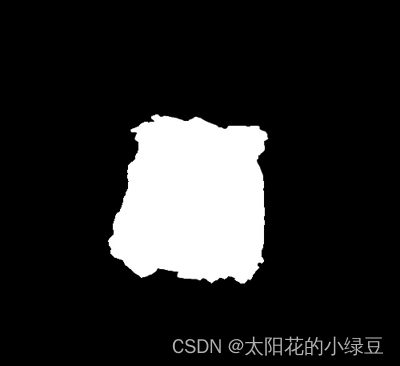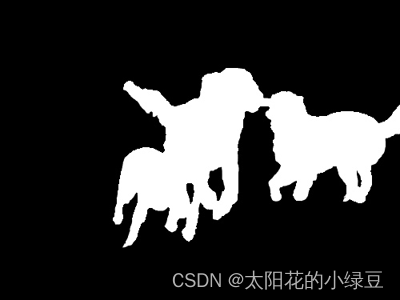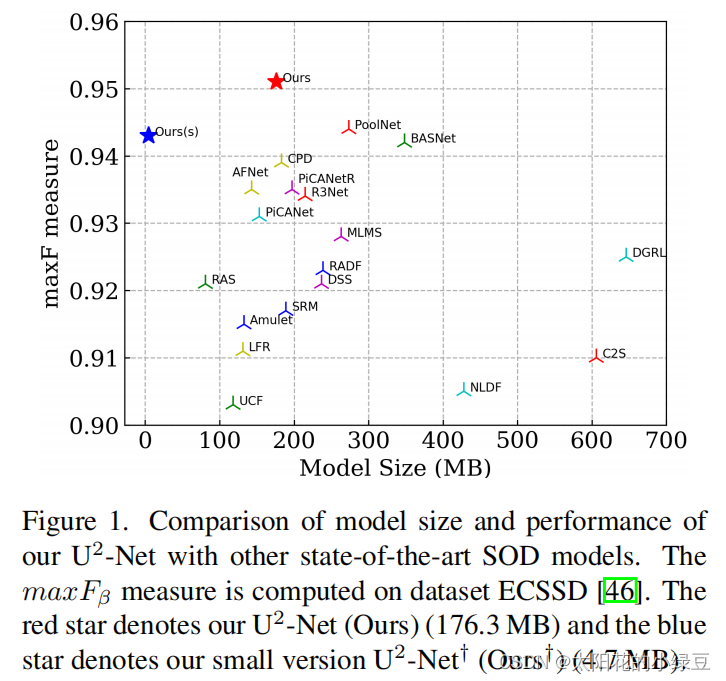## 1 网络结构解析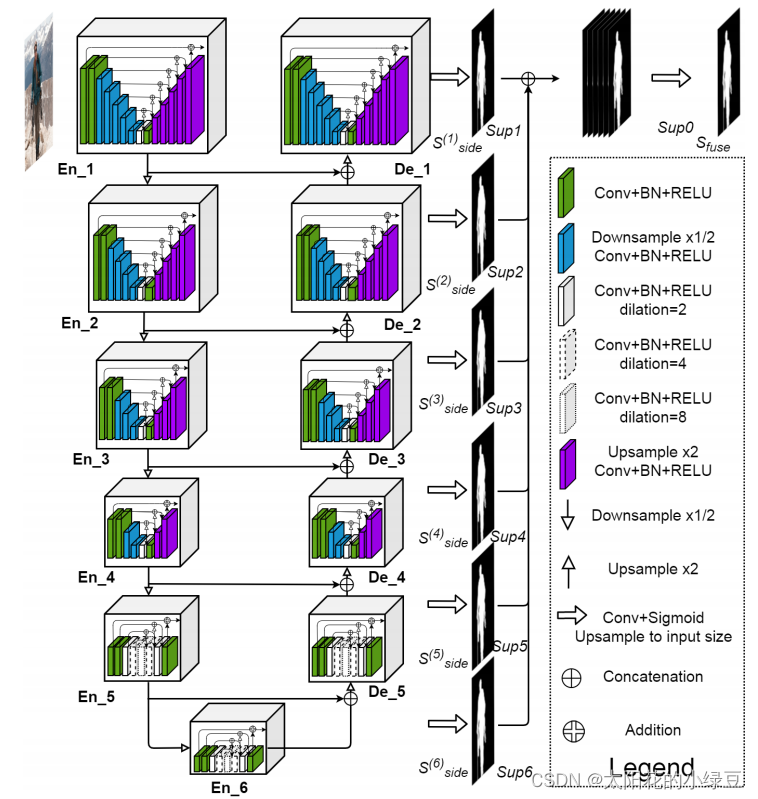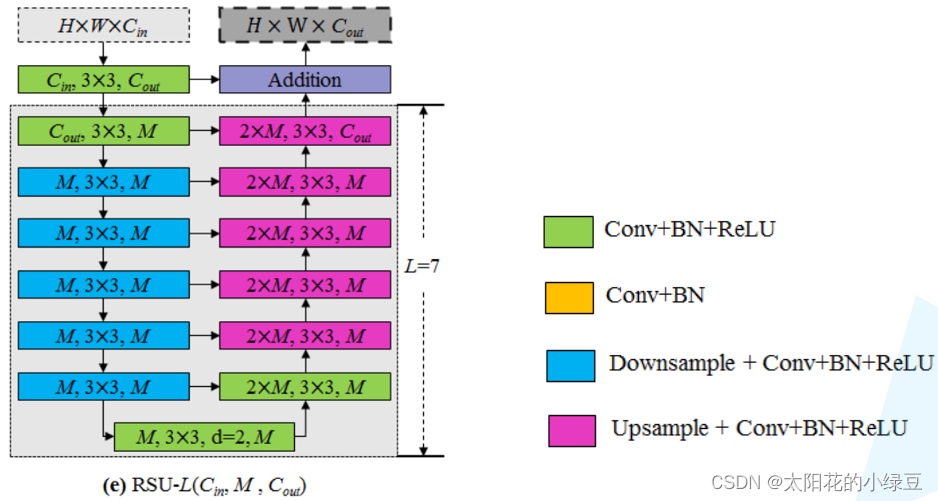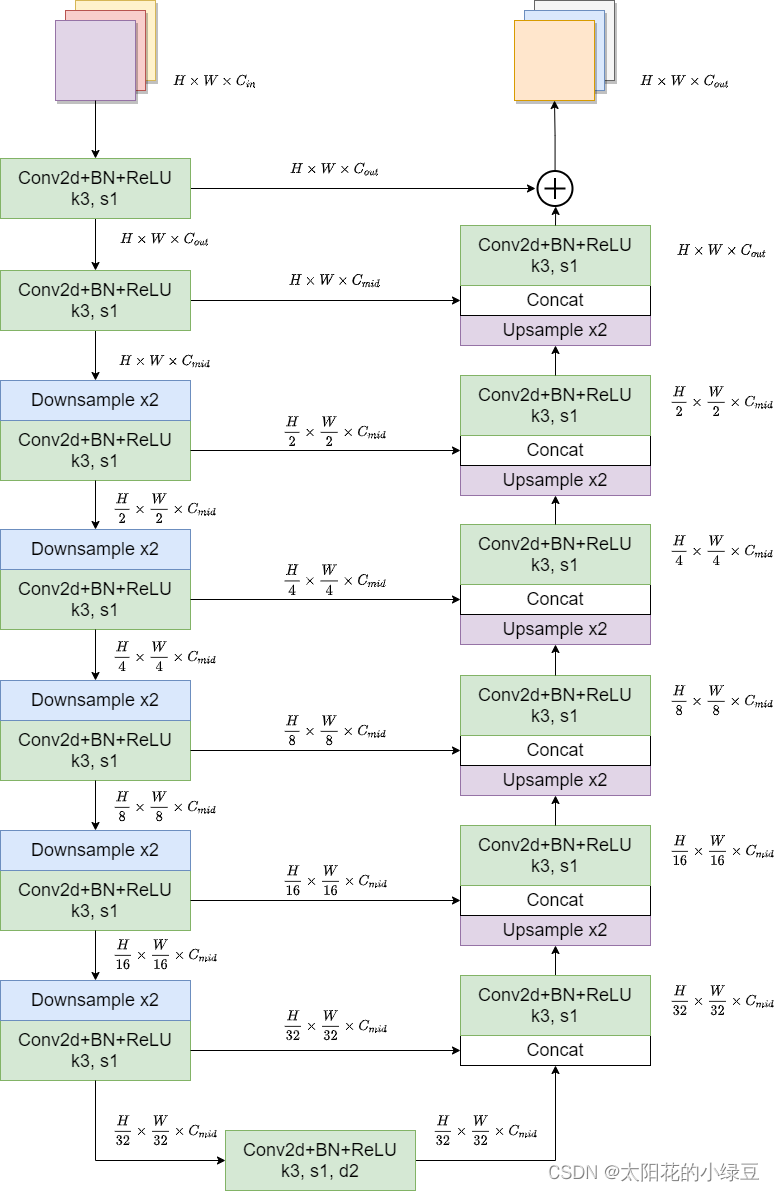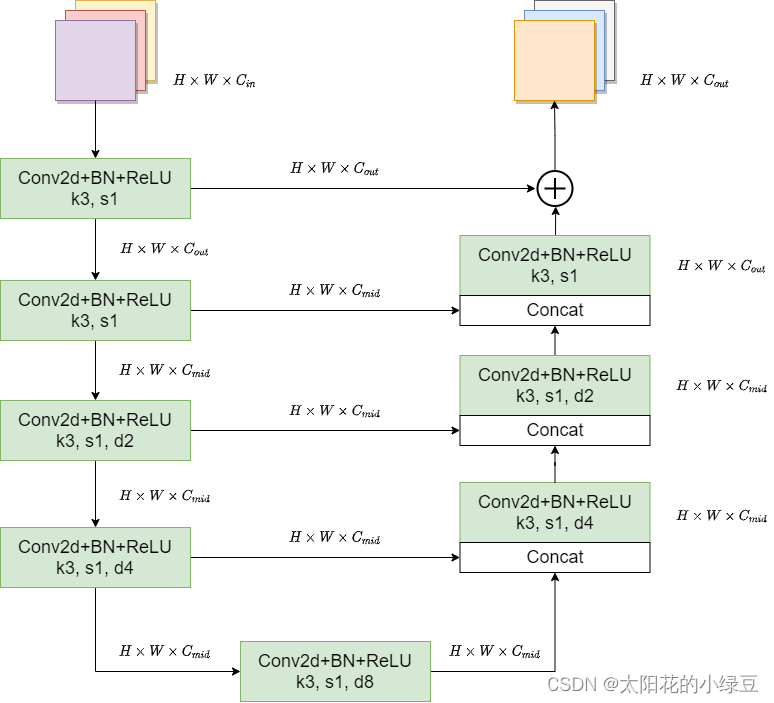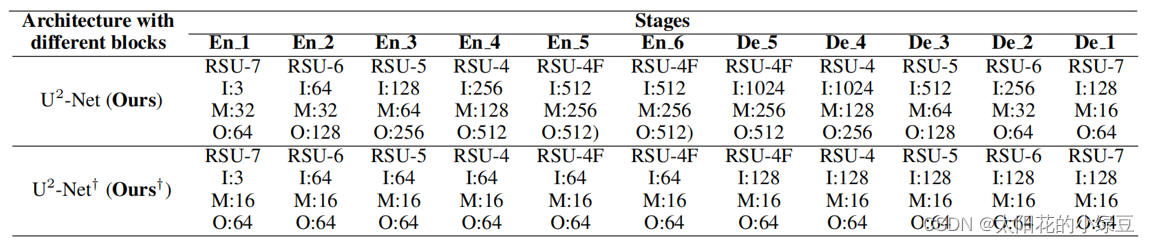## 2 损失计算

L = ∑ m = 1 M w s i d e ( m ) l s i d e ( m ) + w f u s e l f u s e L=\sum_{m=1}^M {w_{side}^{(m)} l_{side}^{(m)}} + w_{fuse}l_{fuse}

## 3 评价指标

F-measure是结合了Precision和Recall的综合指标，计算公式如下：
F β = ( 1 + β 2 ) × P r e c i s i o n × R e c a l l β 2 × P r e c i s i o n + R e c a l l F_{\beta} = \frac{(1 + \beta^2) \times Precision \times Recall}{\beta^2 \times Precision + Recall}

MAEMean Absolute Error的缩写，计算公式如下：
M A E = 1 H × W ∑ r = 1 H ∑ c = 1 W ∣ P ( r , c ) − G ( r , c ) ∣ MAE = \frac{1}{H \times W} {\textstyle \sum_{r=1}^{H}} {\textstyle \sum_{c=1}^{W}} \left | P(r, c) - G(r, c) \right |

## 4 DUTS数据集简介

• DUTS数据集官方下载地址：http://saliencydetection.net/duts/
• 如果下载不了，可以通过我提供的百度云下载，链接: https://pan.baidu.com/s/1nBI6GTN0ZilqH4Tvu18dow 密码: r7k6
• 其中DUTS-TR为训练集，DUTS-TE是测试（验证）集，数据集解压后目录结构如下：
├── DUTS-TR
│      ├── DUTS-TR-Image: 该文件夹存放所有训练集的图片
│
└── DUTS-TE
├── DUTS-TE-Image: 该文件夹存放所有测试（验证）集的图片import os

import cv2
import torch.utils.data as data

class DUTSDataset(data.Dataset):
def __init__(self, root: str, train: bool = True, transforms=None):
assert os.path.exists(root), f"path '{root}' does not exist."
if train:
self.image_root = os.path.join(root, "DUTS-TR", "DUTS-TR-Image")
else:
self.image_root = os.path.join(root, "DUTS-TE", "DUTS-TE-Image")
assert os.path.exists(self.image_root), f"path '{self.image_root}' does not exist."

image_names = [p for p in os.listdir(self.image_root) if p.endswith(".jpg")]
assert len(image_names) > 0, f"not find any images in {self.image_root}."

for p in image_names:

self.images_path = [os.path.join(self.image_root, n) for n in image_names]

self.transforms = transforms

def __getitem__(self, idx):
image_path = self.images_path[idx]
assert image is not None, f"failed to read image: {image_path}"
image = cv2.cvtColor(image, cv2.COLOR_BGR2RGB)  # BGR -> RGB
h, w, _ = image.shape

if self.transforms is not None:
image, target = self.transforms(image, target)

return image, target

def __len__(self):
return len(self.images_path)

if __name__ == '__main__':
train_dataset = DUTSDataset("/data/DUTS", train=True)
print(len(train_dataset))

val_dataset = DUTSDataset("/data/DUTS", train=False)
print(len(val_dataset))

i, t = train_dataset


10553
5019